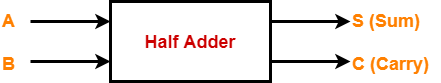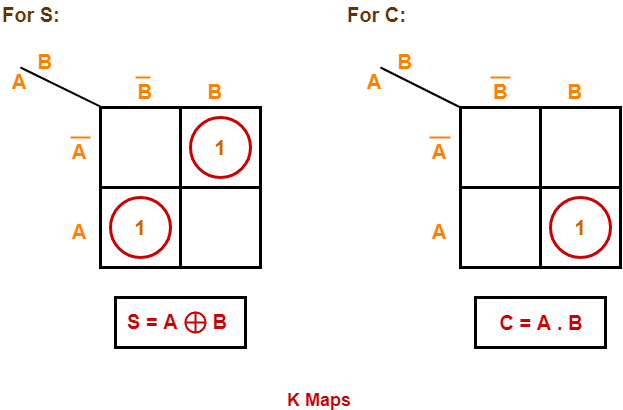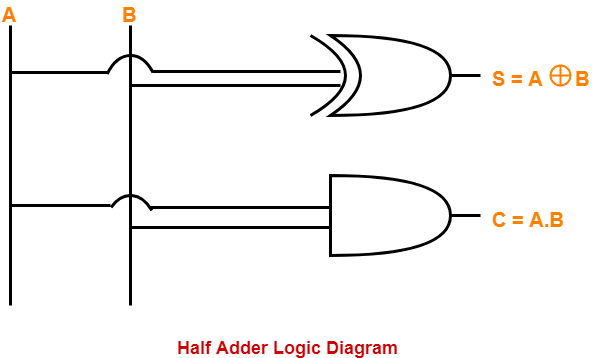## Half Adder | Definition | Circuit Diagram | Truth Table

• Half Adder is a combinational logic circuit.
• It is used for the purpose of adding two single bit numbers.
• It contains 2 inputs and 2 outputs (sum and carry).Half adder is designed in the following steps-

### Step-01:

Identify the input and output variables-

• Input variables = A, B (either 0 or 1)
• Output variables = S, C where S = Sum and C = Carry

### Step-02:

Draw the truth table-

 Inputs Outputs A B C (Carry) S (Sum) 0 0 0 0 0 1 0 1 1 0 0 1 1 1 1 0

### Step-03:

Draw K-maps using the above truth table and determine the simplified Boolean expressions-### Step-04:

Draw the logic diagram.

The implementation of half adder using 1 XOR gate and 1 AND gate is as shown below-• Half adders have no scope of adding the carry bit resulting from the addition of previous bits.
• This is a major drawback of half adders.
• This is because real time scenarios involve adding the multiple number of bits which can not be accomplished using half adders.

To overcome this drawback, Full Adder comes into play.